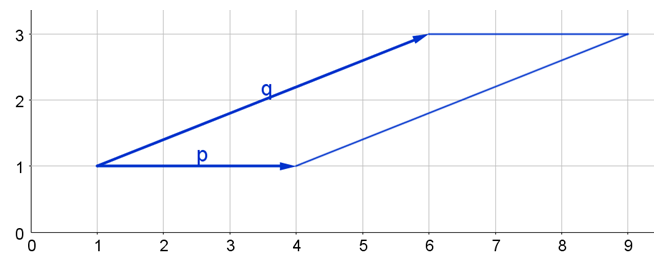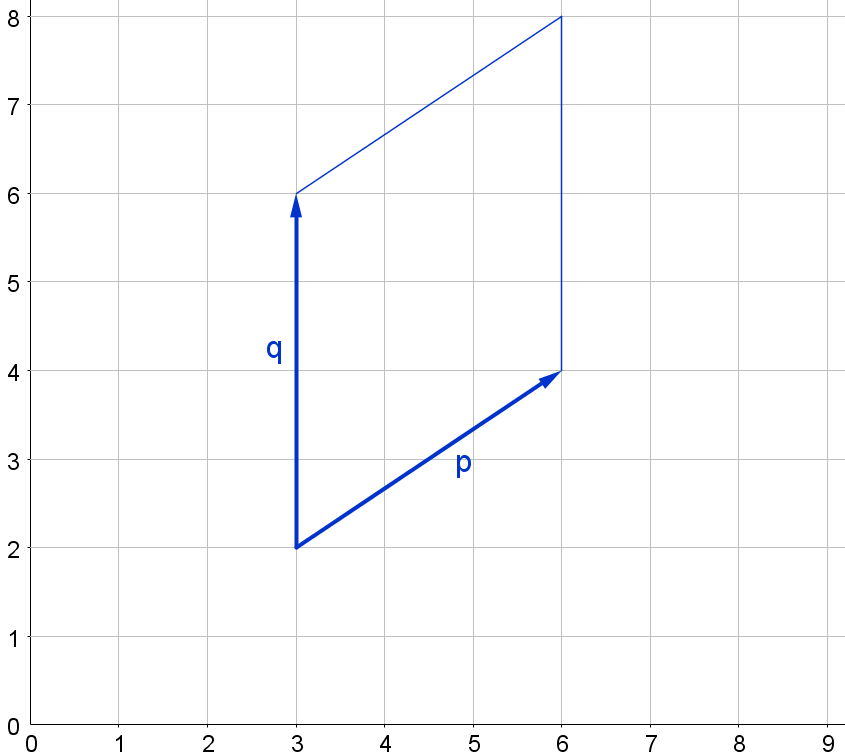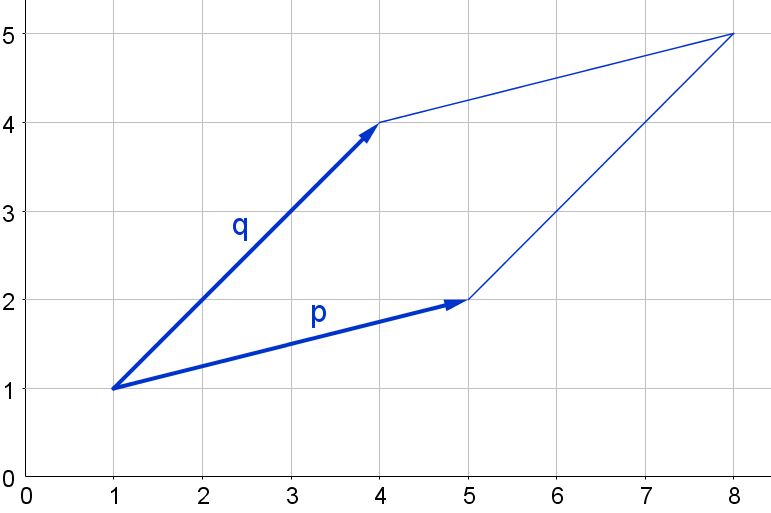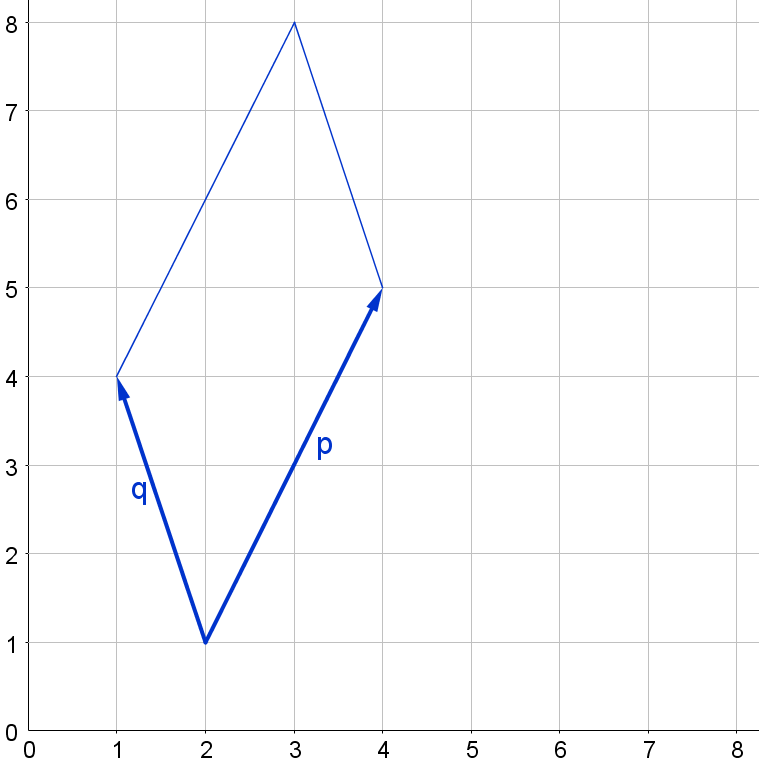#### You may also like### At a Glance

The area of a regular pentagon looks about twice as a big as the pentangle star drawn within it. Is it?### Six Discs

Six circular discs are packed in different-shaped boxes so that the discs touch their neighbours and the sides of the box. Can you put the boxes in order according to the areas of their bases?### Equilateral Areas

ABC and DEF are equilateral triangles of side 3 and 4 respectively. Construct an equilateral triangle whose area is the sum of the area of ABC and DEF.

# Areas of Parallelograms

##### Age 14 to 16Challenge Level
Here are two parallelograms, defined by the vectors $\mathbf{p}$ and $\mathbf{q}$. Can you find their areas?

a) $\mathbf{p}=\left(\begin{array}{c}3\\ 0\end{array}\right), \mathbf{q}=\left(\begin{array}{c}5 \\ 2\end{array}\right)$b) $\mathbf{p}=\left(\begin{array}{c}3 \\ 2\end{array}\right), \mathbf{q}=\left(\begin{array}{c}0 \\ 4\end{array}\right)$Choose different vectors $\mathbf{p}$ and $\mathbf{q}$, where one vector is parallel to an axis, and find the areas of the corresponding parallelograms.
Can you discover a quick way of doing this?

Here are two more parallelograms, again defined by vectors $\mathbf{p}$ and $\mathbf{q}$. This time, neither $\mathbf{p}$ nor $\mathbf{q}$ is parallel to an axis.
Can you find the areas of these parallelograms?

c) $\mathbf{p}=\left(\begin{array}{c}4 \\ 1\end{array}\right), \mathbf{q}=\left(\begin{array}{c}3 \\ 3\end{array}\right)$d) $\mathbf{p}=\left(\begin{array}{c}2 \\ 4\end{array}\right), \mathbf{q}=\left(\begin{array}{c}-1 \\ 3\end{array}\right)$Choose some other vectors p and q, where neither p nor q is parallel to an axis.

Can you find a quick way of working out the areas of the corresponding parallelograms?

Can you find the area of the parallelogram defined by the vectors $\mathbf{p}=\left(\begin{array}{c}a \\ b\end{array}\right)$ and $\mathbf{q}=\left(\begin{array}{c}c \\ d\end{array}\right)$?

If you have found a rule, does it ever give you negative areas?
If so, can you predict which vector pairs have this effect?